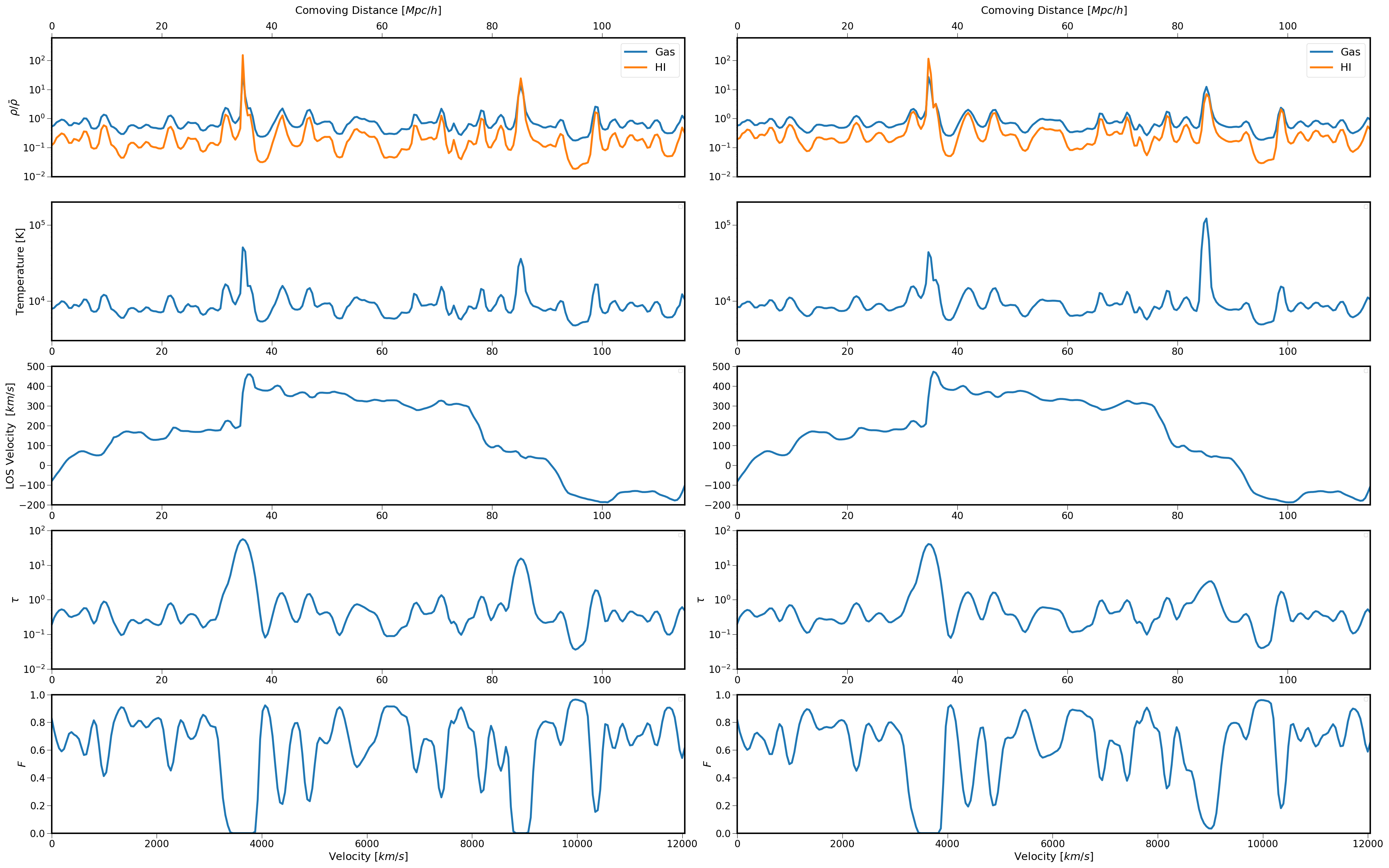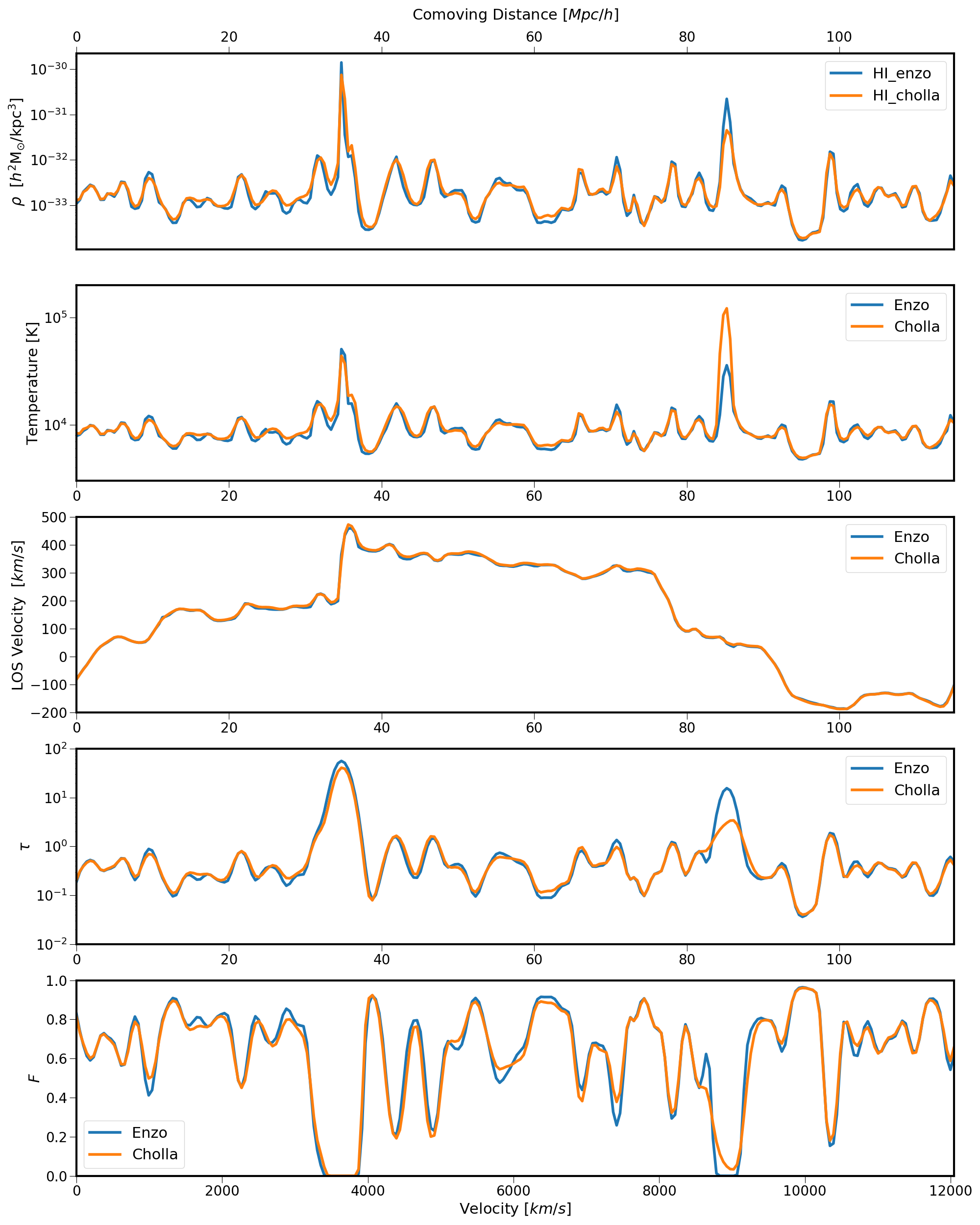Here is spectra generated from the same line of sight on Enzo ( left ) and on Cholla ( right ).

The redshift is: $z=2.35$

The absorption lines are computed using:

where $\Delta v_{\mathrm{D}}=(b / c) v_{0}$ is the Doppler width, using the Doppler parameter $b=b_{\text { thermal }}=\sqrt{2 k_{\mathrm{B}} T / m_{\mathrm{H}}}$.Here is a better comparison: